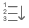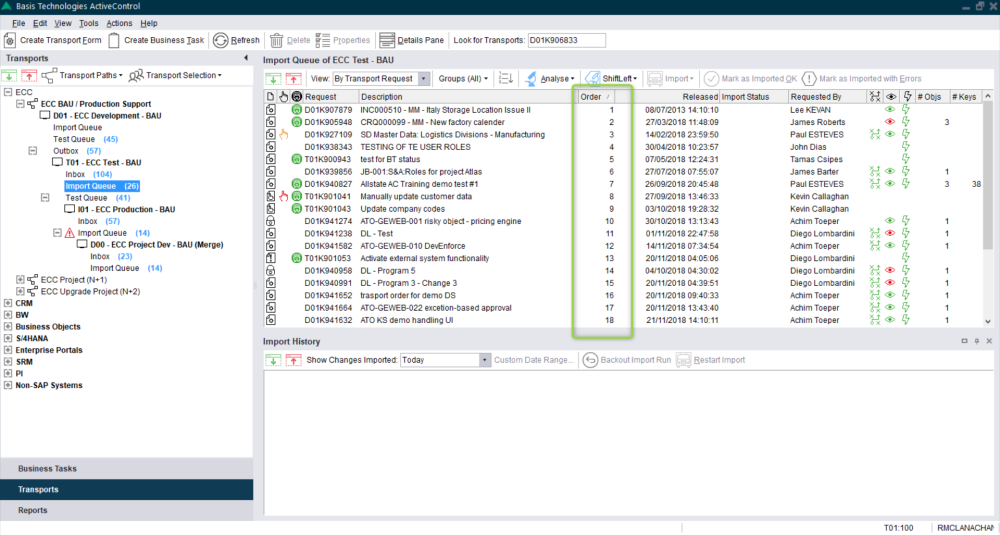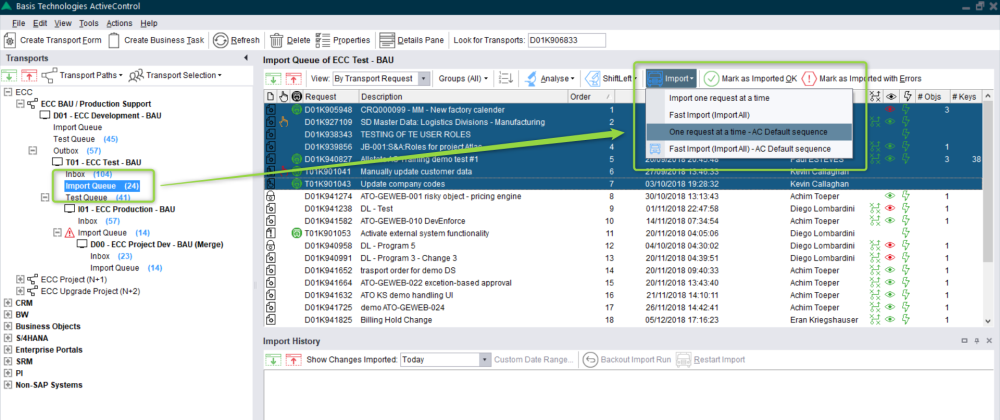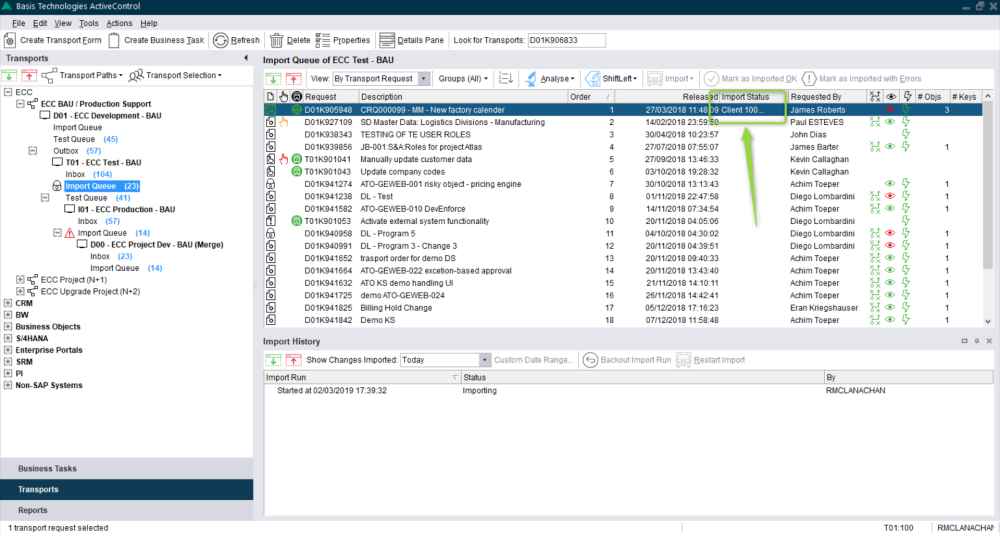Only the Transports waiting in an import queue can be imported by ActiveControl. Once approved and waiting in an import queue, ActiveControl will:

• Allow the transports to be manually imported (only by users authorised to do so)
• Automatically import all waiting transports via a scheduled import job

For automatic imports the sequence will be calculated as follows:

1. The order in which the transports were released.
2. If dependencies are set, then this will override the release order and observe the dependencies between transports.
3. If the target system has been configured to import in the same sequence as a preceding system this will also override the release order. For example, regression systems are set to follow the same import sequence as test systems and production systems will follow the same import sequence as regression systems.

To view the ActiveControl calculated sequence, there are two methods:

1. Use thebutton to sort them. This is only available if the screen sequence is not already in the ActiveControl calculated sequence.

2. Click on the ‘Order’ column to sort them. If this is not shown by default, highlight the required import queue and select “Choose Columns”:Figure: Sequencing in the Windows GUI, via [Order] column

Note: There is no Order column when viewing by project or task. In this case ActiveControl will maintain the order within the task. Tasks and projects are approved in the sequence they are required, ActiveControl will always give alerts in the event that items are going to result in a sequence issue.

ActiveControl will record your preferences so the ‘Order’ only needs to be selected once for each view and then it will remain in place.

To manually import a selection of transports, highlight the ones to be imported and choose either:

• Import one request at a time: This imports the transports one by one in the order selected
• Fast import – Import All: This uses the SAP Import All function to import all transports at the same time. This is useful where there are circular dependencies between transports (e.g. Data Dictionary objects used across transports)
• One request at a time – AC default sequence: This imports the transports one by one in the ActiveControl calculated sequence even if the screen sequence is different. ActiveControl pre-sorts the transports prior to import
• Fast Import (Import All) – AC default sequence: This imports the transports using the block import method in the ActiveControl calculated sequence even if the screen sequence is different. ActiveControl pre-sorts the transports prior to import.Figure: Import Methods within ActiveControl

When ActiveControl is performing the import the transports will be queued with the current importing one highlighted with the client it is being imported into:Figure: Transport being imported in the Windows GUI